[BACK]Computer Modeling in Engineering & SciencesDOI: 10.32604/cmes.2022.020412

ARTICLE

Accelerated Iterative Learning Control for Linear Discrete Systems with Parametric Perturbation and Measurement Noise

1School of Energy and Architecture, Xi'an Aeronautical University, Xi'an, 710077, China
2School of Automation, Northwestern Polytechnical University, Xi'an, 170072, China
*Corresponding Author: Saleem Riaz. Email: saleemriaznwpu@mail.nwpu.edu.cn
Received: 22 November 2021; Accepted: 31 December 2021

Abstract: An iterative learning control algorithm based on error backward association and control parameter correction has been proposed for a class of linear discrete time-invariant systems with repeated operation characteristics, parameter disturbance, and measurement noise taking PD type example. Firstly, the concrete form of the accelerated learning law is presented, based on the detailed description of how the control factor is obtained in the algorithm. Secondly, with the help of the vector method, the convergence of the algorithm for the strict mathematical proof, combined with the theory of spectral radius, sufficient conditions for the convergence of the algorithm is presented for parameter determination and no noise, parameter uncertainty but excluding measurement noise, parameters uncertainty and with measurement noise, and the measurement noise of four types of scenarios respectively. Finally, the theoretical results show that the convergence rate mainly depends on the size of the controlled object, the learning parameters of the control law, the correction coefficient, the association factor and the learning interval. Simulation results show that the proposed algorithm has a faster convergence rate than the traditional PD algorithm under the same conditions.

Keywords: Iterative learning control; monotone convergence; convergence rate; gain adjustment

1  Introduction

The system has gradually become one of the most highly debated research topics in the field of control in recent years . The system is a type of important hybrid system that consists of a set of differential equations, finite differences, and switching rules that change based on actual environmental factors, enabling the whole system to switch between different subsystems to adapt to the demands of different conditions on the system and improve system performance . Therefore, the system is widely used in practical engineering systems, such as traffic control systems , power systems, circuit systems , network control systems , etc. At present, many research results related to the system are focused on the system's stability , but the research results on the output tracking control of the system are very limited . The reason is that the tracking control of the system is much more difficult to achieve than the stabilization and stability problem.

Iterative learning control  has a simple structure, does not require specific model parameters, and can make the behavior of the executed object meet the expected requirements only after enough iterations in a limited interval. This learning algorithm has been widely employed in the control of rigid robot arms , batch processing in the process industry , aerodynamic systems , traffic control systems , electrical and power systems, and other areas due to the characteristics as mentioned earlier . However, most scholars focus on non-system control problems, and research on system iterative learning control problems is limited .

In industrial applications, the controlled system parameters are usually time-varying, so the classical PID and combined PID-like control schemes are particularly inflexible when dealing with the system with uncertain factors . In addition, the analysis and design process of some existing modern control schemes  is complex and difficult. The designed control algorithm and structure should be simple enough and easy to implement to solve these problems. The control scheme should contain the characteristics of nonlinearity, robustness, flexibility and learning ability. With the rapid development of intelligent control technology to solve the uncertainty and complexity of the controlled object, some neural network models and neural network training schemes have been applied to the design of system controllers . For example, as a feedforward controller, Plett  discussed how neural networks learn to imitate the inverse of the controlled object. However, the neural network has the disadvantages of slow learning speed and weak generalization ability, and there is no systematic method to determine its topology. Suppose there is not a timely manner sable control and compensation. In that case, the system noise and random interference will appear in the input end of the controller, which will greatly reduce the stability of the adaptive process and seriously affect the control accuracy. Adaptive filtering has been widely developed [21,22], and neural network is the most commonly used in all kinds of nonlinear filtering. However, it is highly nonlinear in terms of parameters .

Above mentioned scholars are studying the model uncertainty in different fields such as model prediction, system identification , fault detection , motor control , and nonlinear control . Still, there is no specific control algorithm for satisfactory fast error convergence and specific to consider the system coupling, uncertainty, time-varying characteristics, measurement noise and other factors. Adaptive control strategy is proposed in these literature  which can compensate at some extent. An adaptive control is mainly used to deal with complex nonlinear systems with unknown parameters. Based on Lyapunove stability theory, parameter novelty law is designed to achieve system stabilization and progressive tracking of target trajectory [33,34]. Both some special nonlinear systems linearized to parameters [35,36] and nonlinear systems with general structures  have achieved remarkable development. For systems that cannot be modeled or contain un-modeled states, literature [38,39] proposed the model-free adaptive control theory. However, these adaptive control methods cannot solve the problem of complete tracking over a finite time interval .

This paper emphases on a class of discrete time-invariant arbitrary systems that perform repeating tracking tasks on an expected trajectory in a finite time interval based on the above analysis. A PD type is taken as an example, under the condition that the switching sequence is randomly determined, and the iteration is unchanged, by applying characteristics of iterative learning control, provide a discrete iterative learning control algorithm with error backward association to correct control quantity of the next iteration. Combined with the theory of hypervector and spectral radius, the algorithm's convergence is discussed, and sufficient conditions for the algorithm's convergence are given theoretically.

The article can be divided in different sections in order to demonstrate the contribution briefly. The Main contribution and results are comprised in the following sections. Problem formulation is briefly described in Section 2. The convergence analysis, theory of hypervector, spectral radius, and the sufficient conditions for the error convergence are elaborated in Section 3. Then the following Section 4 has showed the numerical example for the validity of the proposed algorithm. Finally, the results summarization of this paper is described in Section 5.

2  Problem Formulation

Consider the following class of linear discrete time-invariant single input and single output systems with repetitive parameter perturbation and measurement noise over a finite period:

{xk(t+1)=(A+ΔA(t)xk(t)+(B+ΔB(t))uk(t))yk(t+1)=Cxk(t+1)+nk(t+1)(1)

where t{0,1,,N1}, NZ+, a subscript k is the number of iterations, xk(t)Rn, uk(t)R, yk(t)R are the state, input and output of the system, respectively. The A, B and C are constant matrices of the corresponding dimension satisfying the condition. nk(t+1)R is the measurement noise of the system, ΔA(t) and ΔB(t) is the uncertainty matrix of the system and the uncertainty input matrix at the time t, such that

ΔA(t)=E1P(t)F1ΔB(t)=E2Q(t)F2

Here, (E1,F1) and (E2,F2) are constant matrices of the corresponding dimension satisfying the condition, define the structure of the uncertain state matrix and the uncertain input matrix; P(t) and Q(t) are unknown matrices, satisfying PT(t)P(t)I and QT(t)Q(t)I.

In the iterative learning process of the system (1), the expected trajectory is set as yd(t+1), and the iteration is unchanged, the corresponding expected state is xd(t), and the corresponding predicted control input is ud(t), Following assumptions are made:

Assumption 1. In each system iteration, the initial state is equal to the ideal initial state, i.e., xk(0)=xd(0).

Assumption 2. Expects trajectory yd(t+1)t{0,1,,N1} is given in advance, independent of the number of iterations.

Assumption 3. For any given desired trajectory yd(t+1), there is an expected state yd(t) and an expected control signal ud(t), so that

{xd(t+1)=Axd(t)+Bud(t)yd(t+1)=Cxd(t+1),t{0,1,N1}(2)

Basing on system (1), define A¯=A+ΔA(t), B¯=B+ΔB(t), output signal of k iteration at time [1,N] could be represented as

yK(1)=Cxk(1)+nk(1)=CA¯xk(0)+CB¯uk(0)+nk(1)yK(2)=Cxk(2)+nk(2)=CA¯2xk(0)+CA¯B¯uk(0)+CB¯uk(1)+nk(1)yK(N)=Cxk(N)+nk(N)=CA¯Nxk(0)+CA¯N1B¯uk(0)+CA¯N2B¯uk(1)++CB¯uk(N1)+nk(N)(3)

For ease of description, write the above expression in the form of a hypervector, and introduce a hypervector:

Uk=[uk(0),uk(1),,uk(N1)]TYk=[yk(1),yk(2),,yk(N)]TNk=[nk(1),nk(2),,nk(N)]T

The above equation can be description as

Yk=(G+ΔG)Uk+Vxk(0)+nk(4)

where

G=[CB000CABCB00CA2BCABCB0CAN1BCAN2BCABCB].

ΔG=[CΔB(0)000CΔB(1)00CΔB(2)0CΔB(N1)].

V=[CA¯,CA¯2,CA¯3,CA¯N]T

*represents an uncertain value by the dynamics and uncertain parameters of the system (1).

System (1), under the condition that assumption 1–3 satisfied, considers a control rule of error backward association and subsequent control quantity correction:

The correction of the error before time t to the control quantity at the current time t

u~k(t)=uk(t)+Li=0tek(i+1)eK(it+1N)2,t{0,1,,N1}(5a)

The learning control rule of PD type iterative as

uk+1(t)=u~k(t)+βek(t)+γek(t+1)(5b)

where uk+1(t) is the control quantity at time t of the k+1th iteration, uk(t) is the control quantity at time t of the kth iteration, u~k(t) is the correction of the control quantity at time t in the kth iteration. β is the proportional gain of PD type learning rule, and γ is the differential gain of PD type learning rule. ek(t+1)=yd(t+1)yk(t+1) is defined as the tracking error. The goal of iterative learning control is to find a control signal sequence {uk(t)} through a certain learning algorithm, so that the output trajectory yk(t+1) of the controlled system (1) under the control of this sequence can converge to the expected trajectory yd(t+1) asymptotically with the increasing number of iterations, namely limk|ek(t+1)|=0, t{0,1,,N1}.

The correction of control quantity (5a) is explained in detail below, as shown in Fig. 1. In the learning process of the kth iteration, the error ek(1) at point 1 will correct the control quantity of N moments in the process of the k+1th iteration, and the correction amount is shown in Table 1.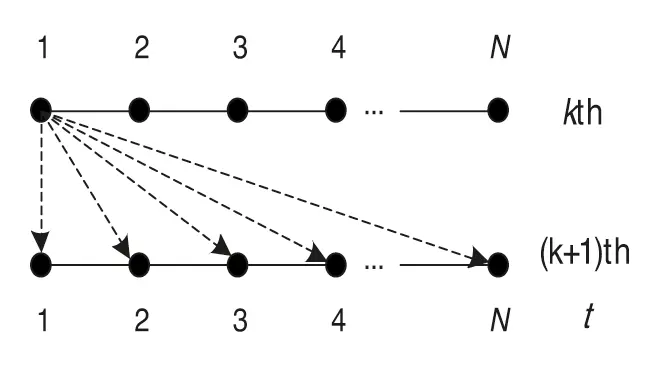Figure 1: ek(1) corrected the control quantity at a certain time in the process of the k+1 iteration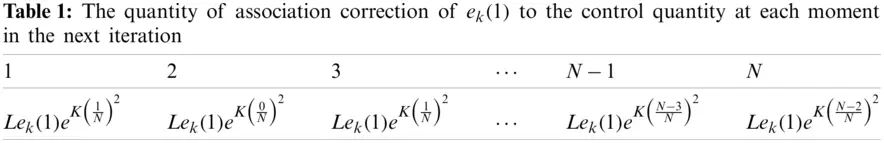ek(2) at point 2 corrected the control quantity of N1 moments in the process of k+1th iteration, as shown in Fig. 2. The correction quantity is shown in Table 2.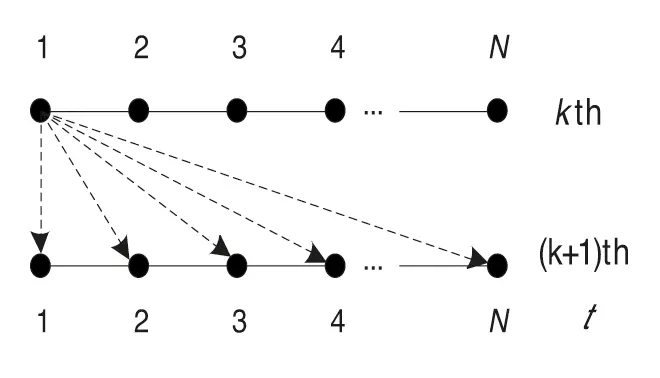Figure 2: ek(2) at point 2 corrected the control quantity of N1 moments in the process of the k+1 iterationAccording to this method, up to the point, its error is ek(N) and it only corrects the control quantity of moment N in the k+1 iteration as shown in Fig. 3. The correction quantity is Lek(N)eK(1N)2.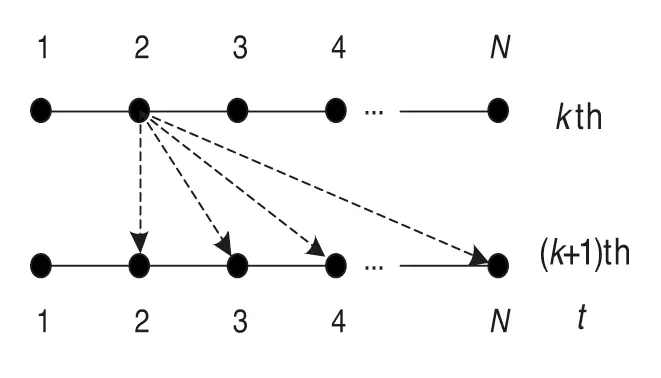Figure 3: ek(N) will correct the control quantity of moment N in the k+1 iteration

According to the above analysis, the correction quantity of each error to the control quantity of following moments can be plotted. The correction u~k(t) is the accumulation of the correction for all previous moments (see Table 3), as

u~k(0)=uk(0)+Lek(1)eK(1N)2u~k(1)=uk(1)+Lek(1)eK(0N)2+Lek(2)eK(1N)2u~k(2)=uk(2)+Lek(1)eK(1N)2+Lek(2)eK(0N)2+Lek(3)eK(1N)2u~k(N1)=uk(N1)+Lek(1)eK(N2N)2+Lek(2)eK(N3N)2++Lek(N)eK(1N)2(6)

which is consistent with Eq. (5a).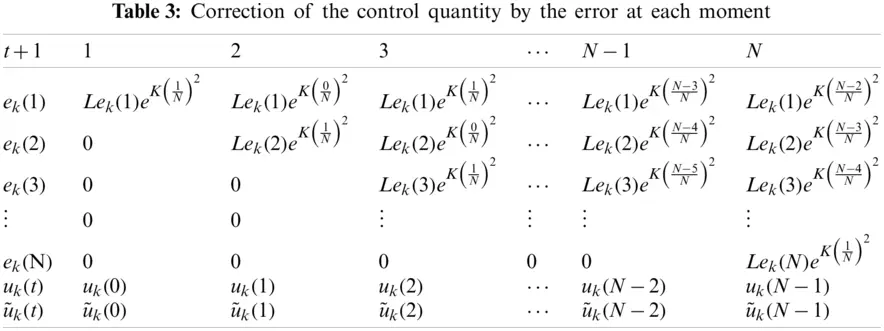3  Convergence Analysis

Lemma 1 Let Ak, BCn×n,(k=0,1,2,), limk+Ak=B, then limk+||Ak||=||B||.

Proof With triangle inequality of norm, 0|||Ak||||B|||||AkB||, when.k+, limk+Ak=B, so limk+||AkB||=0, from the squeeze criterion limk+|||Ak||||B|||=0, so limk+||Ak||=||B||, where |||| is the norm of matrices on Cn×n. Particularly, when limk+Ak=0, we have limk+||Ak||=0.

Lemma 2 Let ACn×n, if limk+Ak=0, then A is called convergent matrix, the necessary and sufficient condition of its convergence is ρ(A)<1.

Proof Necessity. Let A to be a convergency matrix, because of properties spectral radius, (ρ(A))k=ρ(Ak)||Ak|| where |||| is the norm of matrices on Cn×n, So limk+(ρ(A))k=0 and ρ(A)<1.

Sufficiency. Since ρ(A)<1, there exists a positive number ϑ, such that ρ(A)+ϑ<1, Therefore, there exists a norm of matrices on Cn×n say ||||m, such that ||Am||ρ(A)+ϑ<1. Since ||Ak||m||A||mk it can imply that limk+||Ak||m=0, so limk+Ak=0.

3.1 Case of a Determined Model Without Measurement Noise

Theorem 1 Consider a linear discrete time-invariant system (1) with single-input and single-output. If assumption 1–3 is satisfied, the system model is determined, and no measurement noise is obtained; ΔB(t)=0, nk=0, When PD-type accelerated iterative learning control algorithm (5) with association correction is adopted, if the selected learning parameter matrix satisfies

ρ=|1[LeK(1N)2+γ]CB|<1,t{0,1,,N1}(7)

Then the output trajectory uniformly converges to the expected trajectory, that is, when k, yk(t+1)yd(t+1), t{0,1,,N1}.

Proof According to the iterative learning control algorithm (5), in the k+1 iteration, the control quantity at each moment in the interval [0,N1] can be represented as

uk+1(0)=uk(0)+Lek(1)eK(1N)2+γek(1)uk+1(1)=uk(1)+L[ek(1)eK(0N)2+ek(2)eK(1N)2]+γek(2)+βek(1)uk+1(2)=uk(2)+L[ek(1)eK(1N)2+ek(2)eK(0N)2+ek(3)eK(1N)2]+γek(3)+βek(2)

uk+1(N1)=uk(N1)+L[ek(1)eK(N2N)2+ek(2)eK(N3N)2++ek(N1)eK(0N)2+ek(N)eK(1N)2]+γek(N)+βek(N1)

If we introduce the following hypervector

Ek=[ek(1),ek(2),ek(N)]T

Then we have

UK+1=UK+(LH+Γ)Ek(8)

where,

Γ=[γβγβγβγβγ]

Since the model is determined and has no measurement noise, i.e., ΔA(t)=0, ΔB(t)=0, nk = 0, Eq. (4) can be written as Yk=GUk+Vxk(0). Combining xk+1(0)=xk(0)=xd(0) (assumption 1) and Eq. (8), the` error sequence can be derived as

Ek+1=YdYk+1=Yd(GUk+1+Vxk+1(0))=YdG[Uk+(LH+Γ)Ek]Vxk+1(0)=YdGUkVxk(0)G(LH+Γ)Ek=EkG(LH+Γ)Ek=[IN×NG(LH+Γ)]Ek=[IN×NG(LH+Γ)]2Ek1==[IN×NG(LH+Γ)]k+1E0

According to Lemma 2, the necessary and sufficient condition of limk[IN×NG(LH+Γ)]k+1=0 is ρ[IN×NG(LH+Γ)]=maxi|λi|<1, where ρ(M) is the spectral radius of M, λi are eigenvalues of IN×NG(LH+Γ). IN×NG(LH+Γ) is a lower triangle matrix as follows:

IN×NG(LH+Γ)=[1[LeK(1N)2+γ]CB1[LeK(1N)2+γ]CB1[LeK(1N)2+γ]CB]

It is easy to know that the necessary and sufficient condition for the convergence of the system is

ρ=|1[LeK(1N)2+γ]CB|<1,t{0,1,,N1}.

The theorem is proved. I would like to explain this further about the convergence which is basically shows the output within the finite time interval t[0,T] but for different iterations. For instance, as the number of iterations, k, the system's tracking error tends to zero. The system's output after the input update tries to follow within, finite time interval as specified for this system t[0,T]. Ultimately a perfect desired trajectory yd is achieved. The result of the system simulation is shown for the different iterations in simulation section. When the tracking error converges after 15 or more number of iterations and tends to zero, the system's output precisely follows the desired trajectory yd. So it shows that the proposed algorithm is robust and satisfies ρ¯<1 and klim||ek+1()||P=0 is accurate.

3.2 Case of the Undetermined Model without Measurement Noise

Theorem 2 Consider a linear discrete time-invariant system (1) with single-input and single-output. If assumption 1–3 is satisfied, the system model is uncertain but there is no measurement noise, i.e., ΔA(t)0, ΔB(t)0, nk=0. When PD-type accelerated iterative learning control algorithm (5) with association correction is adopted, if the selected learning parameter matrix satisfies

ρ2=maxt|1[LeK(1N)2+γ]CB¯|<1,t{0,1,,N1}(9)

Then the output trajectory uniformly converges to the expected trajectory, that is, when k, yk(t+1)yd(t+1), t{0,1,,N1}, where B¯=B+ΔB(t).

Proof The control rule Eq. (8) is still available. Since the system model is determined and there is no measurement noise, i.e., ΔA(t)0, ΔB(t)0, nk=0. Eq. (4) can be written as Yk=(G+ΔG)Uk+Vxk(0). Combining xk+1(0)=xk(0)=xd(0) (assumption 1) and Eq. (8), the error sequence can be derived as

Ek+1=YdYk+1=Yd[(G+ΔG)Uk+1+Vxk+1(0)+nk+1] =Yd(G+ΔG)[Uk+(LH+Γ)Ek]Vxk+1(0)nk+1 =Yd(G+ΔG)UkVxk(0)nk(G+ΔG)(LH+Γ)Ek+nknk+1 =Ek(G+ΔG)(LH+Γ) Ek+nknk+1 =[IN×N(G+ΔG)(LH+Γ)]Ek+nknk+1

According to Lemma 2, the necessary and sufficient condition of limk[IN×N(G+ΔG)(LH+Γ)]k+1=0 is ρ[IN×N(G+ΔG)(LH+Γ)]=maxi|λi|<1 where ρ(M) is the spectral radius of M, λi are eigenvalues of matrix IN×N(G+ΔG)(LH+Γ). The matrix IN×N(G+ΔG)(LH+Γ) is a lower triangular matrix as follows:

IN×N(G+ΔG)(LH+Γ)=[1[LeK(1N)2+γ]CB¯1[LeK(1N)2+γ]CB¯1[LeK(1N)2+γ]CB¯]

The necessary and sufficient condition for the convergence of the system is

ρ2=maxt|1[LeK(1N)2+γ]CB¯|<1,t{0,1,,N1}

The theorem is proved.

3.3 Case of the Determined Model with Measurement Noise

If the model is determined and has measurement noise, i.e., ΔA(t)=0, ΔB(t)=0, nk0. Eq. (4) can be written as Yk=GUk+V+nk. Combining xk+1(0)=xk(0)=xd(0) (assumption 1) and Eq. (8), the error sequence can be derived as

Ek+1=YdYk+1=Yd[(GUk+1+Vxk+1(0))+nk+1] =YdG[Uk+(LH+Γ)Ek]Vxk+1(0)nk+1 =YdGUkVxk(0)nkG(LH+Γ)Ek+nknk+1 =EkG(LH+Γ) Ek+nknk+1 = [IN×NG(LH+Γ)]Ek+nknk+1

Let P=IN×NG(LH+Γ), then we have Ek+1=PEk+nknk+1.

When k=0, E1=PE0+n0n1;

When k=1, E2=PE1+n1n2=P2E0+(n1n2)+P(n0n1);

When k=2, E3=PE2+n2n3=P3E0+(n2n3)+P(n1n2)+P2(n0n1);

Ek+1=Pk+1E0+i=0kPki(nini+1)(10)

For the repetitive perturbation, ni+1ni=0(i=0,1,2,), Ek+1=[IN×NG(LH+Γ)]k+1E0, according to Lemma 2, the necessary and sufficient condition of limkPk+1=0 is ρ(P)=maxi|λi|<1. ρ(M) represents the spectral radius of matrix M, and λi are eigenvalues of matrix IN×NG(LH+Γ). Refer to the proving process of Theorem 1, it can be obtained that the necessary and sufficient condition of the system convergence is

ρ3=|1[LeK(1N)2+γ]CB¯|<1,t{0,1,,N1}

When k, the system output uniformly converges to the expected trajectory, Hence. ||EK+1||0.

For non-repetitive perturbations, assume that the two-interval perturbations are bounded; there is a positive real number ε< so that the perturbations in the two iterations satisfy ||nk+1nk||ε<.

Theorem 3 Consider a linear discrete time-invariant system (1) with single input and a single output. If assumption 1–3 is satisfied, and there is non-repetitive measurement noise nk(t+1). When PD-type accelerated iterative learning control algorithm (7) with association correction is adopted, if the selected learning parameter matrix satisfies

ρ4=|1[LeK(1N)2+γ]CB|<1,t{0,1,,N1}(11)

Then the system's output converges to a certain neighbourhood of the expected trajectory, that is, when k, ||EK+1||mε, t{0,1,,N1}.

Proof Eq. (10) is still valid. For the non-repeatable perturbations, there is a positive real number ε< for the perturbations in the two iterations satisfy ||nk+1nk||ε<.

Take the norm of both sides of Eq. (10)

||Ek+1||||Pk+1||||E0||+i=0k||Pki||ε

If ρ(P)=maxi|λi|<1, according to Lemmas 1 and 2, limkPk + 1=0, limk||Pk + 1||=0. Define m=i=0k||Pk - i||, since limk||Pk + 1||=0, m< is bounded. Thus, we can the above inequality as ||EK+1||mε.

According to above analysis, the sufficient condition for system convergence is

ρ4=|1[LeK(1N)2+γ]CB|<1

and the error will converge to a boundary, which is mε.

The Theorem 3 is proved.

3.4 Case of Undetermined Model with Measurement Noise

If the system model is not determined and contains measurement noise, that is, ΔA(t)0, ΔB(t)0, nk0, Eq. (4) can be written as Yk=(G+ΔG)Uk+Vxk(0)+nk. Combined with Eq. (8), the error sequence can be derived as

Ek+1=YdYk+1=Yd[(G+ΔG)Uk+1+Vxk+1(0)+nk+1]=Yd(G+ΔG)[Uk+(LH+Γ)Ek]Vxk+1(0)nk+1=Yd(G+ΔG)UkVxk(0)nk(G+ΔG)(LH+Γ)Ek+nknk+1=Ek(G+ΔG)(LH+Γ)Ek+nknk+1=[IN×N(G+ΔG)(LH+Γ)]Ek+nknk+1

Define W=IN×N(G+ΔG)(LH+Γ), then we have Ek+1=WEk+nknk+1

When k=0, E1=WE0+n0n1;

When k=1, E2=WE1+n1n2=W2E0+(n1n2)+W(n0n1);

When k=2, E3=WE2+n2n3=W3E0+(n2n3)+W(n1n2)+W2(n0n1).

Ek+1=Wk+1E0+i=0kWki(nini+1)(12)

For the repetitive perturbation nk+1nk=0, Ek+1=[IN×N(G+ΔG)(LH+Γ)]k+1E0, according to Lemma 2, the necessary and sufficient condition of limkPk+1=0 is ρ(P)=maxi|λi|<1. ρ(M) represents the spectral radius of matrix M, and λi are eigenvalues of matrix IN×N(G+ΔG)(LH+Γ), it can be obtained that the necessary and sufficient condition of the system convergence

ρ5=maxt|1[LeK(1N)2+γ]CB¯|<1,t{0,1,,N1}

For non-repetitive perturbations, assume that the two interval perturbations are bounded, that is, there is a positive real number ε<, so that the perturbations in the two iterations satisfy ||nk+1nk||ε<. In all cases, it is assumed that the initial condition for nk is nk(0)=0.

Theorem 4 Consider a linear discrete time-invariant system (1) with single-input and single-output. If assumption 1–3 is satisfied, and there is non-repetitive measurement noise nk(t+1). When PD-type accelerated iterative learning control algorithm (7) with association correction is adopted, if the selected learning parameter matrix satisfies

ρ6=maxt|1[LeK(1N)2+γ]CB¯|<1,t{0,1,,N1}(13)

Then the output of the system converges to A certain neighborhood of the expected trajectory, that is, when k, ||EK+1||pε, t{0,1,,N1}.

Proof Eq. (13) still holds, and take the norm to both sides of the equation

||Ek+1||||Wk+1||||E0||+i=0kWkiε

If ρ(W)=maxt|λi|<1, according to Lemmas 1 and 2,limkW(K+1)=0, so limk||W(K+1)||=0. Define p=i=0k||WKi||, since limk||Pk+1||=0, p is bounded, p<. Thus, we can the above inequality as ||EK+1||pε.

According to above analysis, the sufficient condition for system convergence is

ρ6=maxt|1[LeK(1N)2+γ]CB¯|<1,t{0,1,,N1}

and the error will converge to a boundary, which is pε. Theorem 4 is proved.

Depiction on people's association thinking, this paper proposes a new type of association iterative learning control algorithm, which, with the help of kernel function (a monotonically decreasing function), uses the information of the present time to make prediction and correction of the future control input in the current iterative process. The information of the current time corrects the subsequent unlearned time, the closer the current time, the greater the influence, the smaller the opposite. Obviously, the kernel function makes the association iterative learning algorithm more reasonable. In the process of theoretical proof of convergence analysis, the kernel function is eliminated, so it is not reflected in the convergence condition. It is proved that the association algorithm and the traditional iterative learning control have the same convergence conditions, but the simulation result of the fifth part of the paper shows that the algorithm does have much better convergence speed than the traditional iterative learning algorithm.

4  Numerical Examples

In order to verify the validity of the associative correction learning rule proposed in this paper, a class of linear discrete time-invariant single-input single-output systems with repetitive parameter perturbation and measurement noise in a finite time period is considered

{xk(t+1)=(A+ΔA(t))xk(t)+(B+ΔB(t))uk(t)yk(t+1)=Cxk(t+1)+nk(t+1)

where: t{0,1,,N1}

A=[100.20.1],B=,C[0.21]

4.1 Case of Determined Model without Measurement Noise

If the system model is determined and there is no measurement noise, i.e., ΔA(t)=0, ΔB(t) = 0, nk=0. According to Theorem 1, the sufficient and necessary condition of system convergence is

ρ=|1[LeK(1N)2+γ]CB¯|<1.

Let the iterative proportional gain β=0.15, the differential gain γ=0.25, the association factor k=2, the correction factor L=0.25, and the discrete time N=40. The calculation results show that

ρ=|1[LeK(1N)2+γ]CB¯|=0.5<1,

satisfies the convergence condition.

If L=0, the above algorithm degenerates to a traditional PD-type iterative learning control algorithm, whose convergence condition is ρ=|1γCB|=0.75<1, ρ<ρ. According to the spectral radius theory, the smaller the radius of convergence, the faster the iterative learning algorithm converges.

The expected trajectory is yd(t+1)=sin(8t/25), t{0,1,,39} and the initial condition xk(0)=0, kZ+, initial control vector u1(1)=0. When applying the accelerated PD-type learning rule proposed in this paper, the variation trend of ||EK|| the first learning iteration to the 50th learning iteration is shown in Fig. 4. The algorithm can ensure ||EK|| converges to 0. Fig. 5 shows the system's output after the first, fourth, seventh and 11th iterations, respectively, and the convergence of the algorithm can be seen in more detail.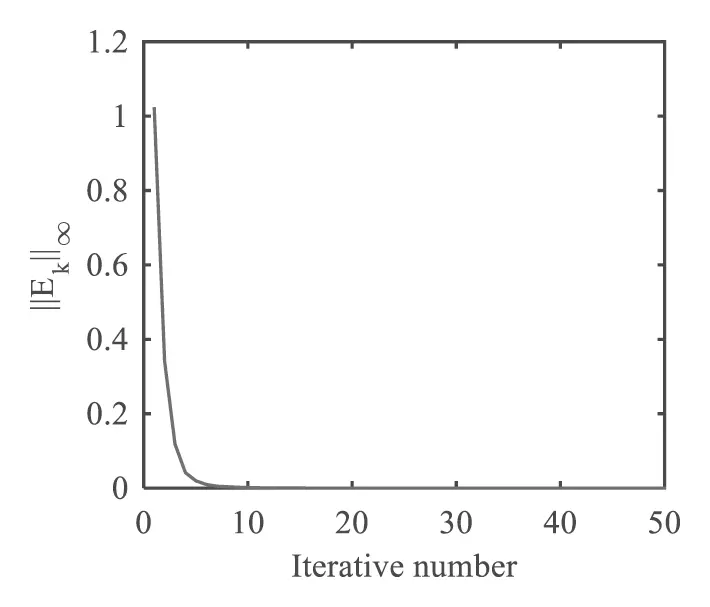Figure 4: The variation trend of the norm of error of accelerated ILC algorithm with the increasing number of iterations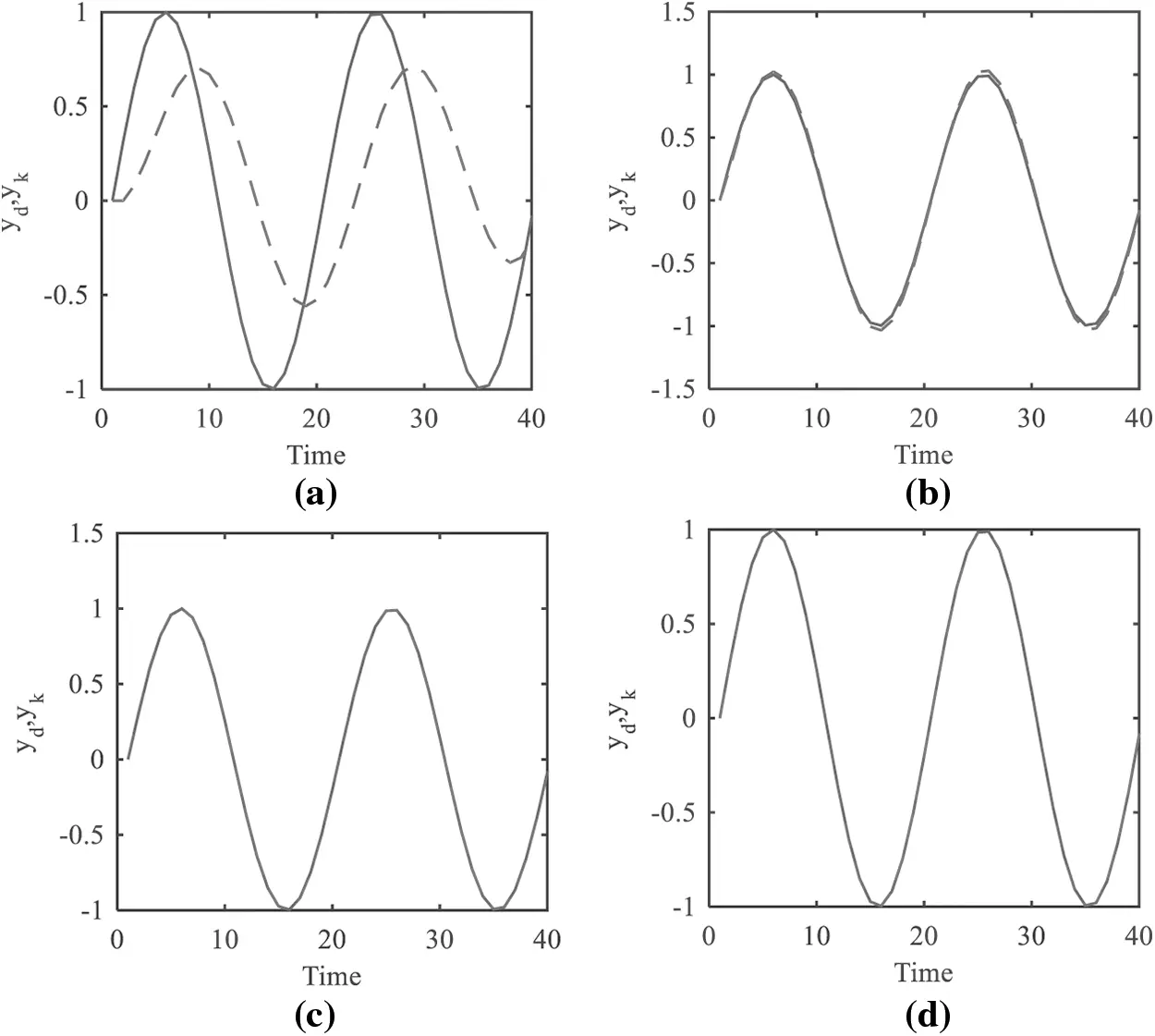Figure 5: Output trajectory and expected trajectory (a) After the first iteration (b) After the fifth iteration (c) After the seventh iteration (d) After the 11th iteration

When the traditional PD-type learning rule is applied, let the iterative proportional gain β=0.15, and the differential gain γ=0.25 remain unchanged during the learning process. From the first learning iteration to the 50th learning iteration, the variation trend of ||EK|| is shown in Fig. 6. In addition, the variation trend of ||EK|| using the acceleration algorithm proposed in this paper is also included in the figure. In the figure, when the allowable error ε=0.01 is given, the traditional PD-type algorithm needs 13 iterations to reach, and the accelerated PD-type iterative learning algorithm needs 6 iterations. Given the permissible error ε=0.001, the conventional PD-type algorithm needs 25 iterations, and the accelerated PD-type iterative learning algorithm needs 11 iterations. It can be intuitively seen that the convergence speed of the system is significantly accelerated after adopting the PD-type accelerated ILC algorithm proposed in this paper.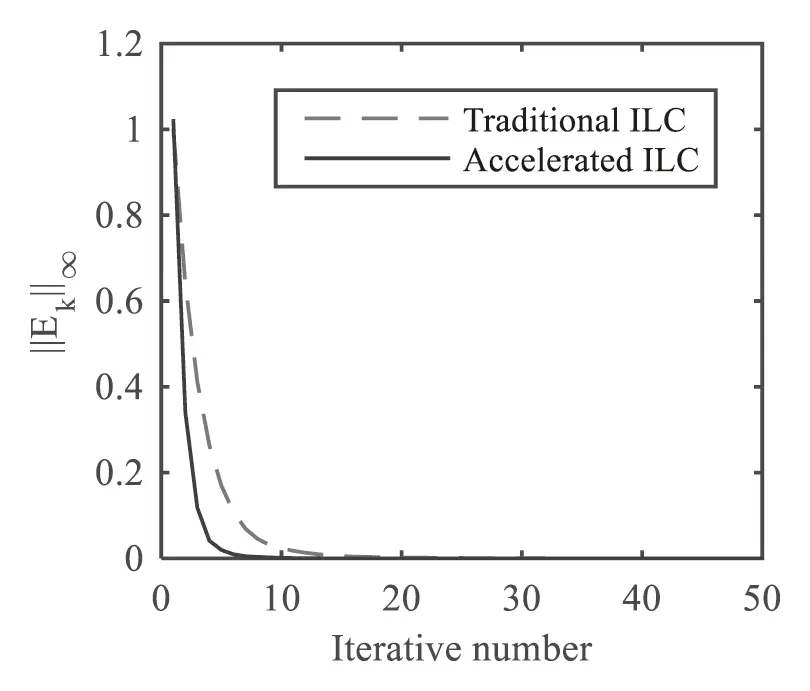Figure 6: Error comparison between the traditional algorithm and the accelerated algorithm

4.2 Case of the Undetermined Model with Measurement Noise

If the system model is not determined and contains measurement noise, that is, ΔA(t)0, ΔB(t)0, nk0. According to Theorem 4, the sufficient condition for the system output to converge to a neighbourhood of the expected trajectory is

ρ6=maxt|1[LeK(1N)2+γ]CB¯|<1,t{0,1,,N1}

The matrix pairs (E1,F1) and (E2,F2) are selected as

E1=[0.2000.2],F1=,E2=[0.1000.1],F2=

Assume P(t) and Q(t) are

P(t)=Q(t)=[Φ1(t)00Φ2(t)]

where |Φi(t)|<1, t{0,1,,N1}, i=1,2. In the simulation process, Φi(t) and Φ2(t) are generated by random function rand(), measurement noise nk=0.08cos(rand()) is randomly generated. The parameters of algorithm (5) are as follows: iterative proportional gain β=0.15, differential gain γ=0.25, association factor k=2, correction factor L=0.25, discrete-time N=40. The result of the simulation indicate that

ρ6=maxt|1[LeK(1N)2+γ]CB¯|=0.4990<1,

meet the convergence condition, while ΔB(t)=(0<0.0013)T.

Expected trajectory is yd(t+1)=sin(8t/25), t{0,1,,39}. The initial xk(0)=0, kZ+, the initial control vector u1(1)=0. When applying the accelerated PD-type learning law proposed in this paper, the changing trend of ||EK||, from the first iteration to the 50th iteration is shown in Fig. 7. The algorithm can ensure ||EK|| converges to 0.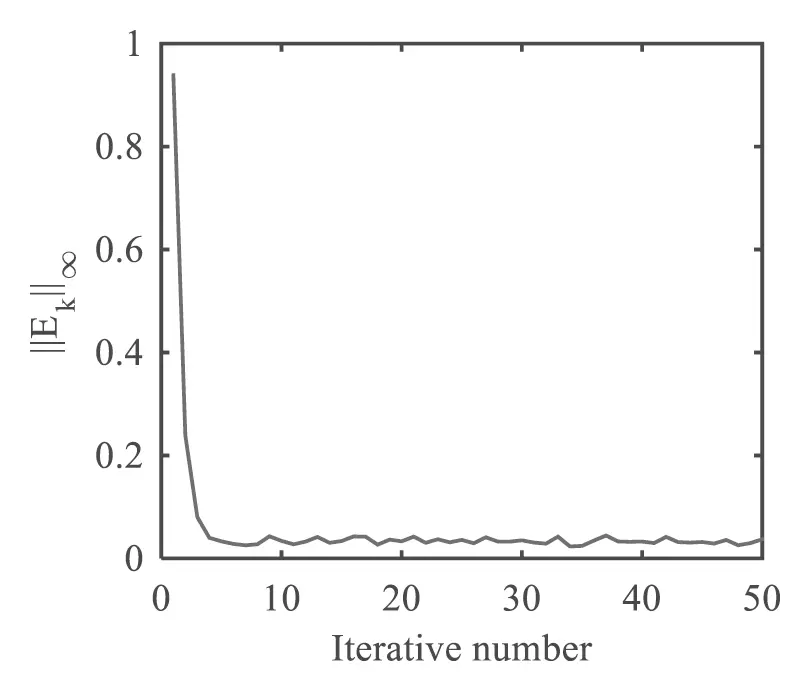Figure 7: The variation trend of the norm of error of accelerated ILC algorithm with increasing number of iterations

In order to observe of the convergence process of the output trajectory, Fig. 8 shows the comparison plots of the system output and the expected trajectory after the first, fourth, seventh and 11th iterations.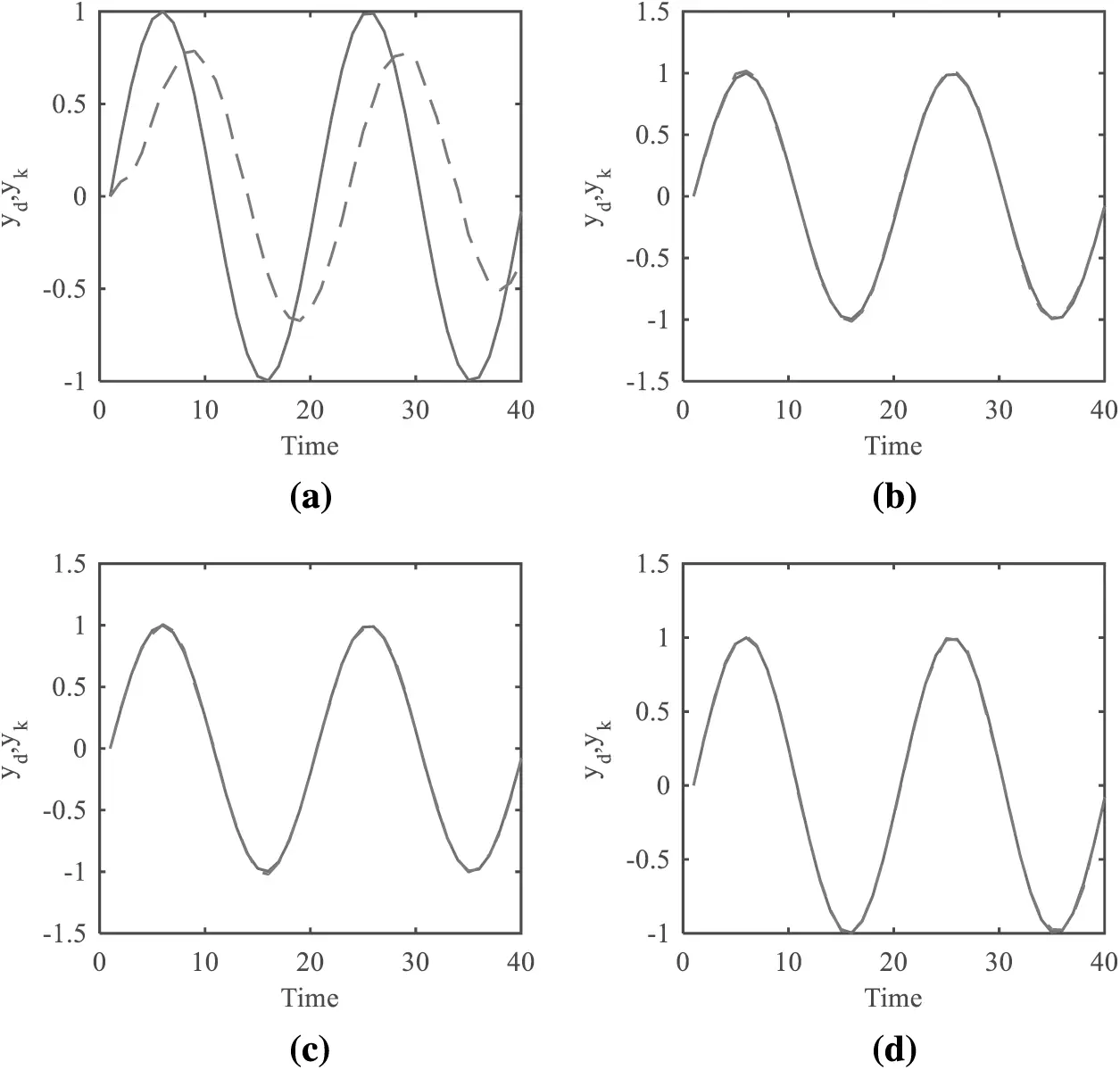Figure 8: Comparison of system output and desired trajectory (a) After the first iteration (b) After the fourth iteration (c) after the 7th iteration (d) after the 11th iteration

If we take L=0, the above algorithm is reduced into the traditional PD type iterative learning control algorithm, the convergence condition for ρ=maxt|1γCB¯|=0.7497<1, and ρ<ρ. According to the spectral radius theory, the smaller the convergence radius is, the faster the iterative learning algorithm converges. Therefore, the association correction iterative learning control algorithm proposed in this paper converges faster. Changing trend of ||EK|| from first iteration to 50th iteration in two algorithms is shown in Fig. 9.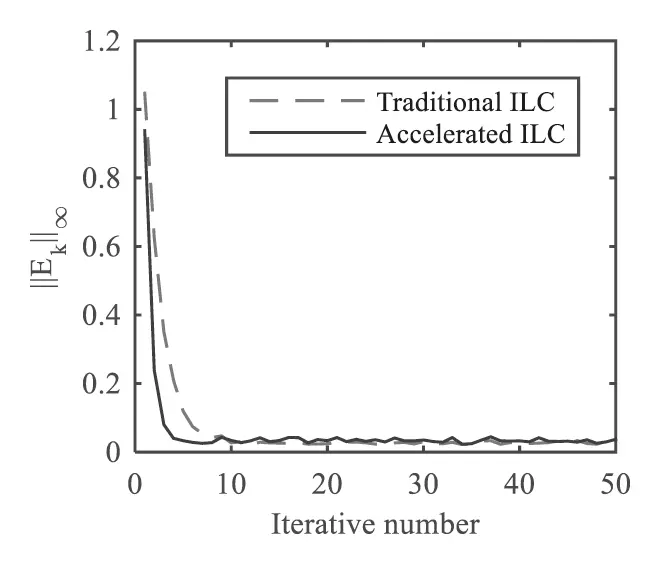Figure 9: Comparison of error convergence rate between traditional PD-type learning rule and accelerated learning rule

It can be seen from the Fig. 9 that the system tracking error does not converge to 0, but to a boundary. Theorem 4, ||EK+1||pε, t{0,1,,N1}. From Fig. 9, it can be intuitively seen that after adopting the PD-type accelerated ILC algorithm proposed in this paper, the convergence speed of the system is significantly increased.

Table 4 shows that the tracking error of P, D, PD and accelerated proposed PD type ILC laws in the first iteration is 1.1217316. After 15th iterations, the error of the P-type law is 0.062823, D type algorithm is 0.07538, and the error of algorithm PD law is 0.024335. Where the error of the proposed accelerated PD law is 0.003683, from the column of Table 4 to the data, the tracking error of all ILC law is reduced consecutively with the increase of iteration number. However, from the horizontal data in Table 4, the tracking error of the proposed accelerated PD law is the smallest as compared to other ILC laws (P, D, PD) under the same iteration number. Therefore, it can be easily observed from Table 4 that the convergence speed of the proposed accelerated PD law in this paper is significantly higher than that of other traditional laws.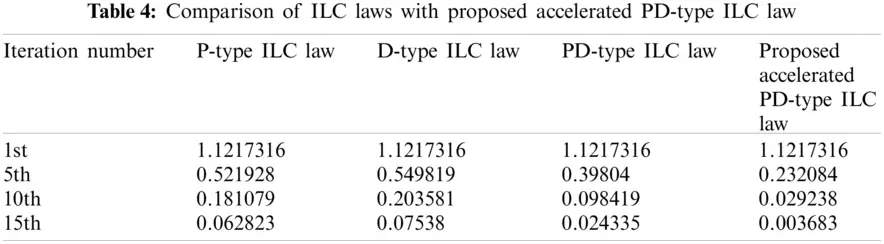The auto-associative ILC proposed in this paper is based on the traditional ILC, namely, using the current information to estimate the future input. Compared to traditional ILC, the new algorithm is characterized as follows: in each trial, the unlearned time is pre-corrected with the current time information. The algorithm can reduce the number of iterations and accelerate the learning convergence speed. The algorithm proposed in this paper differs from the traditional discrete closed-loop algorithm and the higher-order algorithm as follows:

(1)   Although the algorithm proposed in this paper is similar in form to the traditional closed-loop iterative learning algorithm, the principle is completely different from that of the traditional discrete closed-loop PD-type algorithm (feedback algorithm). The traditional discrete closed-loop ILC algorithm is to correct the control input of the current time directly with the error of the previous time in the same trial. The algorithm proposed in this paper uses the error of the current time to pre-estimate the amount of control after which it does not occur at all times, and plays the role of pre-correction.

(2)   Although the associative iterative learning algorithm proposed in this paper is similar in form to the traditional higher-order discrete learning algorithm, the learning process is completely different from the traditional higher-order iterative learning algorithm. The traditional high-order ILC is the algebraic overlay of the control information of the previous two or more trials at the corresponding time. The new iterative learning algorithm proposed in this paper is to pre-correct the subsequent unoccurred time with the error value of the current time in the same trial.

5  Conclusions

The problem of discrete linear time-invariant systems with parameter perturbation and measurement noise is investigated in this paper. It proposes sufficient conditions for convergence of a PD-type accelerated iterative learning algorithm with association correction under the circumstances of parameter determined without measurement noise, parameter undetermined without noise, parameter determined with measurement noise. Parameter undermined with measurement noise, respectively. Under the same simulation conditions, the convergence radius of the proposed algorithm is smaller than that of the traditional PD-type ILC algorithm. The convergence is theoretically proven with the help of hyper vector and spectral radius theory. Numerical simulation shows the effectiveness of the proposed algorithm. The results show that the algorithm can fully track the expected trajectory within finite intervals when uncertain system parameters. In the case of measurement noise existing, the system's output will converge to a neighborhood of the expected trajectory using the algorithm proposed in this paper. In future studies, we will consider the stability and convergence of nonlinear discrete systems with parameter perturbations and measurement noises and the convergence of arbitrary bounded changes of initial conditions.

Acknowledgement: I want to declare on behalf of my co-authors that the work described is original research that has not been published previously and is not under consideration for publication elsewhere, in whole or in part. I confirmed that no conflict of interest exists in submitting this manuscript and is approved by all authors for publication in your journal.

Funding Statement: The authors received no specific funding for this study.

Conflicts of Interest: The authors declare that they have no conflicts of interest to report regarding the present study.

## References

1. Lin, H., & Antsaklis, P. J. (2009). Stability and stabilizability of switched linear systems: A survey of recent results. IEEE Transactions on Automatic Control, 54(2), 308-322. [Google Scholar] [CrossRef]
2. Liberzon, D., & Morse, A. S. (1999). Basic problems in stability and design of switched systems. IEEE Control Systems Magazine, 19(5), 59-70. [Google Scholar] [CrossRef]
3. Balluchi, A., di Benedetto, M. D., Pinello, C., Rossi, C., & Sangiovanni-Vincentelli, A. N. D. A. (1999). Hybrid control in automotive applications: The cut-off control. Automatica, 35(3), 519-535. [Google Scholar] [CrossRef]
4. Wang, X., & Zhao, J. (2013). Partial stability and adaptive control of switched nonlinear systems. Circuits, Systems, and Signal Processing, 32(4), 1963-1975. [Google Scholar] [CrossRef]
5. Yu, J., Wang, L., & Yu, M. (2011). Switched system approach to stabilization of networked control systems. International Journal of Robust and Nonlinear Control, 21(17), 1925-1946. [Google Scholar] [CrossRef]
6. Cheng, D., Guo, L., Lin, Y., & Wang, Y. (2005). Stabilization of switched linear systems. IEEE Transactions on Automatic Control, 50(5), 661-666. [Google Scholar] [CrossRef]
7. Riaz, S., Lin, H., Mahsud, M., Afzal, D., & Alsinai, A. (2021). An improved fast error convergence topology for PDα-type fractional-order ILC. Journal of Interdisciplinary Mathematics, 24(7), 2005-2019. [Google Scholar]
8. Arimoto, S., Kawamura, S., & Miyazaki, F. (1984). Bettering operation of robots by learning. Journal of Robotic Systems, 1(2), 123-140. [Google Scholar] [CrossRef]
9. Liu, J., Wang, Y., Tong, H. T., Han, R. P. (2012). Iterative learning control based on radial basis function network for exoskeleton arm. 2nd International Conference on Advances in Materials and Manufacturing Processes (ICAMMP 2011), vol. 415–417, pp. 116–122. DOI 10.4028/www.scientific.net/AMR.415-417.116. [CrossRef]
10. Sanzida, N., & Nagy, Z. K. (2013). Iterative learning control for the systematic design of supersaturation controlled batch cooling crystallisation processes. Computers & Chemical Engineering, 59, 111-121. [Google Scholar] [CrossRef]
11. Tan, K. K., Lim, S. Y., Lee, T. H., & Dou, H. (2000). High-precision control of linear actuators incorporating acceleration sensing. Robotics and Computer-Integrated Manufacturing, 16(5), 295-305. [Google Scholar] [CrossRef]
12. Hou, Z., Yan, J., Xu, J. X., & Li, Z. (2011). Modified iterative-learning-control-based ramp metering strategies for freeway traffic control with iteration-dependent factors. IEEE Transactions on Intelligent Transportation Systems, 13(2), 606-618. [Google Scholar] [CrossRef]
13. Feng, X., Zhang, Y., Kang, L., Wang, L., & Duan, C. (2021). Integrated energy storage system based on triboelectric nanogenerator in electronic devices. Frontiers of Chemical Science and Engineering, 15(2), 238-250. [Google Scholar] [CrossRef]
14. Ruan, X., & Zhao, J. (2013). Convergence monotonicity and speed comparison of iterative learning control algorithms for nonlinear systems. IMA Journal of Mathematical Control and Information, 30(4), 473-486. [Google Scholar] [CrossRef]
15. Srivastava, S., & Pandit, V. S. (2016). A PI/PID controller for time delay systems with desired closed loop time response and guaranteed gain and phase margins. Journal of Process Control, 37, 70-77. [Google Scholar] [CrossRef]
16. Zhang, J. (2017). Design of a new PID controller using predictive functional control optimization for chamber pressure in a coke furnace. ISA Transactions, 67, 208-214. [Google Scholar] [CrossRef]
17. Chandrakala, K. V., & Balamurugan, S. (2016). Simulated annealing based optimal frequency and terminal voltage control of multi source multi area system. International Journal of Electrical Power & Energy Systems, 78, 823-829. [Google Scholar] [CrossRef]
18. Zhou, S., Chen, M., Ong, C. J., & Chen, P. C. (2016). Adaptive neural network control of uncertain MIMO nonlinear systems with input saturation. Neural Computing and Applications, 27(5), 1317-1325. [Google Scholar] [CrossRef]
19. Patan, K., & Patan, M. (2020). Neural-network-based iterative learning control of nonlinear systems. ISA Transactions, 98, 445-453. [Google Scholar] [CrossRef]
20. Plett, G. L. (2003). Adaptive inverse control of linear and nonlinear systems using dynamic neural networks. IEEE Transactions on Neural Networks, 14(2), 360-376. [Google Scholar] [CrossRef]
21. Afshari, H. H., Gadsden, S. A., & Habibi, S. (2017). Gaussian filters for parameter and state estimation: A general review of theory and recent trends. Signal Processing, 135, 218-238. [Google Scholar] [CrossRef]
22. Hua, Y., Wang, N., & Zhao, K. (2021). Simultaneous unknown input and state estimation for the linear system with a rank-deficient distribution matrix. Mathematical Problems in Engineering, 2021, 11. [Google Scholar] [CrossRef]
23. Feng, X., Li, Q., & Wang, K. (2020). Waste plastic triboelectric nanogenerators using recycled plastic bags for power generation. ACS Applied Materials & Interfaces, 13(1), 400-410. [Google Scholar] [CrossRef]
24. Liu, C., Li, Q., & Wang, K. (2021). State-of-charge estimation and remaining useful life prediction of supercapacitors. Renewable and Sustainable Energy Reviews, 150, 111408. [Google Scholar] [CrossRef]
25. Liu, C. L., Li, Q., & Wang, K. (2021). State-of-charge estimation and remaining useful life prediction of supercapacitors. Renewable & Sustainable Energy Reviews, 150, 17. [Google Scholar] [CrossRef]
26. Liu, J., Ruan, X., & Zheng, Y. (2020). Iterative learning control for discrete-time systems with full learnability. IEEE Transactions on Neural Networks and Learning Systems, 99, 1-15. [Google Scholar] [CrossRef]
27. Zhang, J., & Huang, K. (2020). Fault diagnosis of coal-mine-gas charging sensor networks using iterative learning-control algorithm. Physical Communication, 43, 101175. [Google Scholar] [CrossRef]
28. Zhang, C., & Yan, H. S. (2017). Inverse control of multi-dimensional taylor network for permanent magnet synchronous motor. Compel, 36(6), 1676-1689. [Google Scholar] [CrossRef]
29. Chen, W., Hu, J., Wu, Z., Yu, X., & Chen, D. (2020). Finite-time memory fault detection filter design for nonlinear discrete systems with deception attacks. International Journal of Systems Science, 51(8), 1464-1481. [Google Scholar] [CrossRef]
30. Islam, S. A. U., Nguyen, T. W., Kolmanovsky, I. V., Bernstein, D. S. (2020). Adaptive control of discrete-time systems with unknown, unstable zero dynamics. 2020 American Control Conference (ACC), pp. 1387–1392. IEEE.
31. Xiong, S., & Hou, Z. (2020). Model-free adaptive control for unknown MIMO nonaffine nonlinear discrete-time systems with experimental validation. IEEE Transactions on Neural Networks and Learning Systems, 99, 1-13. [Google Scholar] [CrossRef]
32. Abidi, K., Soo, H. J., & Postlethwaite, I. (2020). Discrete-time adaptive control of uncertain sampled-data systems with uncertain input delay: A reduction. IET Control Theory & Applications, 14(13), 1681-1691. [Google Scholar] [CrossRef]
33. Shahab, M. T., & Miller, D. E. (2021). Adaptive control of a class of discrete-time nonlinear systems yielding linear-like behavior. Automatica, 130, 109691. [Google Scholar] [CrossRef]
34. Riaz, S., Lin, H., & Elahi, H. (2020). A novel fast error convergence approach for an optimal iterative learning controller. Integrated Ferroelectrics, 213(1), 103-115. [Google Scholar] [CrossRef]
35. Liu, L., Liu, Y. J., Chen, A., Tong, S., & Chen, C. P. (2020). Integral barrier lyapunov function-based adaptive control for switched nonlinear systems. Science China Information Sciences, 63(3), 1-14. [Google Scholar] [CrossRef]
36. Riaz, S., Lin, H., Anwar, M. B., & Ali, H. (2020). Design of PD-type second-order ILC law for PMSM servo position control. Journal of Physics: Conference Series, 1707(1), 12002. [Google Scholar] [CrossRef]
37. Moghadam, R., Natarajan, P., Raghavan, K., Jagannathan, S. (2020). Online optimal adaptive control of a class of uncertain nonlinear discrete-time systems. 2020 International Joint Conference on Neural Networks, pp. 1–6. IEEE, Virtual, Glasgow, UK.
38. Chi, R., Hui, Y., Zhang, S., Huang, B., & Hou, Z. (2019). Discrete-time extended state observer-based model-free adaptive control via local dynamic linearization. IEEE Transactions on Industrial Electronics, 67(10), 8691-8701. [Google Scholar] [CrossRef]
39. Li, Y., & Dankowicz, H. (2020). Adaptive control designs for control-based continuation in a class of uncertain discrete-time dynamical systems. Journal of Vibration and Control, 26(21–22), 2092-2109. [Google Scholar] [CrossRef]
40. Hui, Y., Chi, R., Huang, B., Hou, Z., & Jin, S. (2020). Observer-based sampled-data model-free adaptive control for continuous-time nonlinear nonaffine systems with input rate constraints. IEEE Transactions on Systems, Man, and Cybernetics: Systems, 51(12), 7813-7822. [Google Scholar] [CrossRef]This work is licensed under a Creative Commons Attribution 4.0 International License, which permits unrestricted use, distribution, and reproduction in any medium, provided the original work is properly cited.